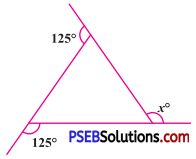# PSEB 8th Class Maths Solutions Chapter 3 Understanding Quadrilaterals Ex 3.2

Punjab State Board PSEB 8th Class Maths Book Solutions Chapter 3 Understanding Quadrilaterals Ex 3.2 Textbook Exercise Questions and Answers.

## PSEB Solutions for Class 8 Maths Chapter 3 Understanding Quadrilaterals Ex 3.2

Question 1.
(a)Solution:
Sum of all the exterior angles of a polygon = 360°.
∴ x + 125° + 125° = 360°
∴ x + 250° = 360°
∴ x = 360° – 250° (Transposing 250° to RHS)
∴ x = 110°

(b)Solution:
In this figure, two exterior angles are of 90° each. (one interior angle is 90°)
Sum of all exterior angles of a polygon = 360°.
∴ x + 90° + 60° + 90° + 70° = 360°
∴ x + 310° = 360°
∴ x = 360° – 310° (Transposing 310° to RHS)
∴ x = 50°Question 2.
Find the measure of each exterior angle of a regular polygon of (i) 9 sides (ii) 15 sides
Solution:
(i) Number of sides (n) = 9
∴ Number of exterior angles = 9
The sum of all exterior angles = 360°.
The given polygon is a regular polygon.
∴ All the exterior angles are equal.
∴ Measure of an exterior angle = $$\frac{360^{\circ}}{9}$$.
= 40°

(ii) Number of sides of regular polygon = 15
∴ Number of exterior angles = 15
The sum of all the exterior angles = 360°
The given polygon is a regular polygon.
∴ All the exterior angles are equal.
∴ The measure of each exterior angle = $$\frac{360^{\circ}}{15}$$ = 24°

Question 3.
How many sides does a regular polygon have if the measure of an exterior angle is 24° ?
Solution:
Regular polygon is equiangular.
Sum of all the exterior angles = 360°
Measure of an exterior angle = 24°
∴ Number of sides = $$\frac{360^{\circ}}{24^{\circ}}$$
The polygon has 15 sides.Question 4.
How many sides does a regular polygon have if each of its interior angles is 165° ?
Solution:
The given polygon is regular polygon.
Each interior angle = 165°
∴ Each exterior angle = 180° – 165° = 15°
∴ Number of sides = $$\frac{360^{\circ}}{15^{\circ}}$$ = 24
The polygon has 24 sides.

Question 5.
(a) Is it possible to have a regular polygon with measure of each exterior angle as 22° ?
Solution:
Each exterior angle = 22°
∴ Number of sides = $$\frac{360^{\circ}}{22^{\circ}}=\frac{180^{\circ}}{11^{\circ}}$$
The number of sides of a regular polygon must be a whole number.
But, $$\frac {180}{11}$$ is not a whole number.
∴ No, exterior angle of a regular polygon cannot be of measure 22°.

(b) Can it be an interior angle of a regular polygon ? Why ?
Solution:
If the measure of an interior angle of a polygon is 22°, then the measure of its exterior angle = 180° – 22° = 158°.
∴ Number of sides = $$\frac{360^{\circ}}{158^{\circ}}=\frac{180^{\circ}}{79^{\circ}}$$
$$\frac {180}{79}$$is not a whole number.
∴ No, 22° cannot be an interior angle of a regular polygon.Question 6.
(a) What is the minimum interior angle possible for a regular polygon? Why ?
Solution:
The minimum number of sides of a polygon = 3
The regular polygon of 3-sides is an equilateral triangle.
Each interior angle of an equilateral triangle = 60°.
Hence, the minimum possible interior angle of a polygon = 60°.

(b) What is the maximum exterior angle possible for a regular polygon ?
Solution:
The sum of an exterior angle and its corresponding interior angle is 180°. (Linear pair)
And minimum interior angle of a regular polygon = 60°.
∴ The maximum exterior angle of a regular polygon = 180° – 60° = 120°.